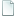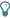Topic: How to Make NWC Play Jazz (Read 13003 times)

## How to Make NWC Play Jazz##### 2002-05-10 03:40 pm
Here is a way to make NWC play jazz - see the *.nwc files I join in jazzy.zip (1K).

In the tempo track (create one if necessary), create a measure containing MPCs (Insert: Multi-point Controller, Tempo) before each half of beat, which changes the tempo. For example, for a 60 "regular" tempo, 1st MPC contains (in absolute value) 45, the 2nd MPC (2nd half beat) contains 120, and so on. Now repeat that 4 times (in 4/4), and repeat the measure (using repeat bars), and this should give a 2/3, 1/3 feeling. You can adjust this precisely to make the 2nd half beat shorter or longer (see below for exact values).

The formula to be used to determine tempo values is :

(T1 = tempo of 1st half beat, T2 = tempo of 2nd half beat, T = normal tempo)
2/3 + 1/3 : T1 = 3/4 * T and T2 = 3/2 * T
3/4 + 1/4 : T1 = 2/3 * T and T2 = 2 * T

The generic formula, for any ratio, is :
T1 = 1/2 * T * (1 + ratio)
T2 = 1/2 * T * (1 + 1/ratio)
where ratio = length (NOT tempo!) of 2nd half beat / length of 1st half beat. For example, for 2/3 + 1/3, ratio = (1/3)/(2/3) = 1/2.

(Many thanks to Andrew to have made me think of this adaptation on the hint I gave on lazy feel...)